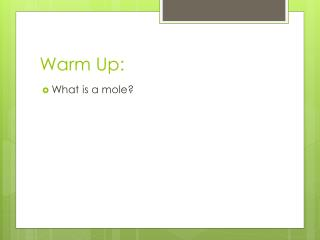Download PresentationWarm Up:

# Warm Up:

Download Presentation## Warm Up:

- - - - - - - - - - - - - - - - - - - - - - - - - - - E N D - - - - - - - - - - - - - - - - - - - - - - - - - - -
##### Presentation Transcript

1. Warm Up: • What is a mole?

2. Chapter 10: The Mole Learning Targets: To form an idea of what a mole is. To form an idea of the relationships between, particles, moles, and mass. Distinguish between parts/mass to mols and parts to mass problems. Be able to calculate: parts to mols/mols to parts mass to mols/mols to mass mass to parts/parts to mass

3. What is a mole? • How many are in a pair? • Dozen? • Gross? • Ream? What do these represent? a specific value

4. What is a mole? • 1 mole = 6.023 x 1023 ____________ • This number is known as Avogadro's Number • It is the number of particles whatever they may be: atoms, molecules, formula units • If I have a mole of apples, how many apples do I have? • A mole of marbles? • A mole of skittles?

5. Avogadro’s Number • 1 mole = 6.023 x 1023___units/particles____ • The units/particles depends on what type of particle it is: • Atoms: individual elements • Formula Units (FU): Ionic Compounds • Molecules: Covalent Compounds

6. Let’s work on determining types of units: • What would the unit of the following be:

7. Converting Moles to Particles • How many atoms are in 2.54 moles of sulfur. __Given Unit_Conversion Factor_(New Unit) Conversion Factor (given unit) What is our conversion factor? 6.02 x 1023 particles (atoms, molecules, formula units)

8. Converting Moles to Parts: • Knowing what we know now: • How many atoms are in 2.45 moles of sulfur? 2.45 mols S 6.022 x 1023atmsS 1mol S =1.48 x 1024atms S

9. Let’s Practice: • How many atoms are in 6.2 moles of Al? • Convert 5.75 x 1024 atoms of Al into mols of Al.

10. A couple more: • How may atoms are in 3.5 moles of Si? • Convert 5.3 x 1025 molecules of CO2 to moles.

11. Now mass to moles: • Which has more mass? A dozen watermelon? A dozen eggs?

12. Mass and the mole: • AMU: (atomic mass unit) the mass of one nucleon (proton and neutron) • Carbon’s AMU: 12 (12 protons and neutrons) • 1 mol = a substance’s AMU • 1 mol of Carbon has an AMU of 12 grams. • AMU is an individual atom/Mole is 6.02 x 1023 atoms • Every mole has a different mass value based on their AMU, because each atom has a different mass.

13. Molar Mass: • To determine the mass of a substance or element. • Use the periodic table to determine the mass of a single mole of a substance. • The molar mass comes from the periodic table. • AMU=molar mass • Unit for molar mass is g/mol

14. What is the molar mass? • Copper: • Aluminum: • Hydrogen: • Nitrogen: • Uranium: • Sulfur: • Oxygen: • Chlorine: • K: • Beryllium:

15. Molar Mass of Compounds: • Add the molar masses of EVERY atom in the compound together. • Find the molar mass of H2O H= 1.00795 x 2 hydrogen atoms 2.02g O=15.994 x 1 oxygen atom + 16.00 g Molar mass of H2O 18.02g/mol *You can use whole numbers instead of decimals but you will need to round!

16. Find the Molar Mass:

17. Moles to grams • First you must find the molar mass of the substance… You will need this to complete the problem. • Then you plug the information into “train tracks” How many moles in 56.71g of water (H2O)? 56.71g H2O 1 mol H2O = 56.71 x 1 = 3.151 mols H2O 18g H2O 18 *Remember the molar mass of H2O is 18g/mol.

18. Let’s Practice: How many grams in 1.56 moles of Calcium Chloride? How many grams can be found in 12.8 moles of Copper (II) Phosphate?

19. Practice: How many moles are in 15.6g of sulfur dioxide? How many moles can be found in 456g of lithium carbonate?

20. Practice: How many mols of sodium is 3g? How many mols of Fe is .05g? How many mols of Beryllium is 1.60g?

21. Practice: How many mols of lead is 15g? How many mols of platinum is 2.3 x 10-5g?

22. Two step problems: • Parts mols grams If there is 2.45 x 1023atms of Calcium how many grams is that? 2.45 x 1023atms Ca 1mol Ca 40 g Ca 6.02 x 1023atms Ca 1 mol Ca

23. Practice If we have 567.1g of gold, how many atoms is that? How many molecules are in 56.7g of Hydrochloric Acid?

24. Practice: What is the mass of 4.56 x 1021 atoms of Mg? How many formula units are in 125.3g of strontium carbonate? What is the mass of 8.65 x 1025 formula units of nickel (III) sulfate?